# Custom Normalize AD Indicator, why it gives zero divide error, yet the print check shows the value is not Zero ?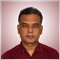502

Hi

I have created a custom indicator to calculate normalized AD. (The base is AD.mq5 from Examples)

It is causing me Zero divide error and when I check values with PrintFormat statement, it is non zero value.

Here is the code ...

```//+------------------------------------------------------------------+
//+------------------------------------------------------------------+
#property copyright   "2009-2020, MetaQuotes Software Corp."

#property indicator_separate_window
#property indicator_buffers 3
#property indicator_plots   2
#property indicator_type1   DRAW_LINE
#property indicator_color1  LightSeaGreen
#include  <MovingAverages.mqh>
//--- input params
input int                 InpAvgPeriod  = 13;
input ENUM_APPLIED_VOLUME InpVolumeType = VOLUME_TICK; // Volume type
//--- indicator buffer
double BufferVolume[];
//+------------------------------------------------------------------+
//| Custom indicator initialization function                         |
//+------------------------------------------------------------------+
void OnInit()
{
//--- indicator digits
IndicatorSetInteger(INDICATOR_DIGITS,0);
//--- indicator short name
IndicatorSetString(INDICATOR_SHORTNAME,"AKT AD% ... Where we CLOSE in range ");
//--- index buffer
SetIndexBuffer(2,BufferVolume,INDICATOR_CALCULATIONS);
//--- set index draw begin
PlotIndexSetInteger(0,PLOT_DRAW_BEGIN,1);
PlotIndexSetInteger(1,PLOT_DRAW_BEGIN,1);
}
//+------------------------------------------------------------------+
//| Accumulation/Distribution                                        |
//+------------------------------------------------------------------+
int OnCalculate(const int      rates_total,
const int      prev_calculated,
const datetime &time[],
const double   &open[],
const double   &high[],
const double   &low[],
const double   &close[],
const long     &tick_volume[],
const long     &volume[],
{
//--- check for bars count
if(rates_total < 2)
return(0); //exit with zero result
//--- get current position
int pos = prev_calculated-1;
if(pos < 0)
pos = 0;
//--- calculate AD with appropriate volumes
if(InpVolumeType == VOLUME_TICK)
else
int i;
if(prev_calculated > 1)
i = prev_calculated-1;
else
i = 0;
for(i; i < rates_total && !IsStopped(); i++)
{
BufferNormAD[i] = SimpleMA(i,InpAvgPeriod,ExtADbuffer) / SimpleMA(i,InpAvgPeriod,BufferVolume);  // This line is causing Zero Divide error
}
//---
return(rates_total);
}
//+------------------------------------------------------------------+
//| Calculating with selected volume                                 |
//+------------------------------------------------------------------+
void CalculateAD(const int rates_total,const int pos,
const double &high[],
const double &low[],
const double &close[],
const long   &volume[])
{
//--- main cycle
for(int i = pos; i < rates_total && !IsStopped(); i++)
{
double MFM = (close[i]-low[i]) - (high[i]-close[i]);	// Money Flow Multiplier
double MFV;						// Money Flow Volume
if(high[i] == low[i])
MFV = 0.0;
else
{
MFV = (MFM / (high[i]-low[i])) * volume[i];
BufferVolume[i] = (double)volume[i];
}
if(i>0)
}
}
//+------------------------------------------------------------------+
```

2021.09.11 09:55:03.639 Ci_AD (US30,M5)  AD -96.0 Volume 288.0 ... AD and Volume array is NON ZERO values

Wish you all a happy weekend.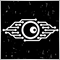327399

Anil Varma -:

Hi

I have created a custom indicator to calculate normalized AD. (The base is AD.mq5 from Examples)

It is causing me Zero divide error and when I check values with PrintFormat statement, it is non zero value.

Here is the code ...

2021.09.11 09:55:03.639 Ci_AD (US30,M5)  AD -96.0 Volume 288.0 ... AD and Volume array is NON ZERO values

Wish you all a happy weekend.

Zero Divide is Zero Divide. You cannot argue against that...

Your problem is in your SimpleMA() function. It is this function that is giving you back the 0 result.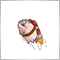3096

Anil Varma -:

Hi

I have created a custom indicator to calculate normalized AD. (The base is AD.mq5 from Examples)

It is causing me Zero divide error and when I check values with PrintFormat statement, it is non zero value.

Here is the code ...

2021.09.11 09:55:03.639 Ci_AD (US30,M5)  AD -96.0 Volume 288.0 ... AD and Volume array is NON ZERO values

Wish you all a happy weekend.
Calling SimpleMa() twice won't create a new instance of the function.502

Flavio Jarabeck #:

Zero Divide is Zero Divide. You cannot argue against that...

Your problem is in your SimpleMA() function. It is this function that is giving you back the 0 result.

Thanks Flavio

So what is way out for this problem?502

Navdeep Singh #:
Calling SimpleMa() twice won't create a new instance of the function.

Thanks Navdeep

I tired creating two separate buffers for each average and then trying to arrive at the average of price / average of volume. Still get the zero divide error, yet the arrays returns a value for both price and volume averages.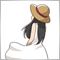893

```//+------------------------------------------------------------------+
//| Simple Moving Average                                            |
//+------------------------------------------------------------------+
double SimpleMA(const int position, const int period, const double &price[])
{
//---
double result = 0.0;
//--- check position
if(position >= period - 1 && period > 0)
{
//--- calculate value
for(int i = 0; i < period; i++) result += price[position - i];
result /= period;
}
//---
return(result);
}
//+------------------------------------------------------------------+```

When "position >= period - 1", MA is calculated. However, in your program, you are starting from 0, so the first return value is "0.0".

Learn how to debug. It's easy to find out by tracing with a debugger.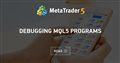Debugging MQL5 Programs
• www.mql5.com
This article is intended primarily for the programmers who have already learned the language but have not fully mastered the program development yet. It reveals some debugging techniques and presents a combined experience of the author and many other programmers.502

When "position >= period - 1", MA is calculated. However, in your program, you are starting from 0, so the first return value is "0.0".

Learn how to debug. It's easy to find out by tracing with a debugger.

Thanks Nagisa

Good to hear from you after long time.

Finally I have got an logical answer to my problem. However, as there was no response from forum I moved to resort to manually calculating SMA instead of using SimpleMA(). I have got results from that only with one issue that it is not showing for MA Period bars. Indicators always gives me hard time :).

I have looked into the article you have suggested and trying learn debugging techniques. Earlier also someone from forum also suggested to use it, but I kept making "Without breakpoints, the debugger will simply execute the program and report that debugging is successful but you will see nothing." mistake and could find it useful so dropped using it.

But the article you have suggested gives clear picture how to use it and I am working to find out errors myself.

Take care and thanks again for your response.893

Anil Varma - #:

I have got results from that only with one issue that it is not showing for MA Period bars. Indicators always gives me hard time :).

Isn't it because of the absence of these lines?

```#property indicator_type1   DRAW_LINE
#property indicator_color1  LightSeaGreen
#property indicator_type2   DRAW_LINE
#property indicator_color2  clrRed```502

When "position >= period - 1", MA is calculated. However, in your program, you are starting from 0, so the first return value is "0.0".

Learn how to debug. It's easy to find out by tracing with a debugger.

Changed the code, still did not work on my PC and noticed that my PC's MovingAverages.mqh is different version !!!

```    int i;
if(prev_calculated > 2)
i = prev_calculated-1;
else
i = 1;
for(i; i < rates_total && !IsStopped(); i++)
{
BufferNormAD[i] = SimpleMA(i,InpAvgPeriod,ExtADbuffer) / SimpleMA(i,InpAvgPeriod,BufferVolume);  // This line is causing Zero Divide error
}```

```//+------------------------------------------------------------------+
//| Simple Moving Average                                            |
//+------------------------------------------------------------------+
double SimpleMA(const int position,const int period,const double &price[])
{
double result=0.0;
//--- check period
if(period>0 && period<=(position+1))	... the code is different on my MovingAverages.mqh file !!!
{
for(int i=0; i<period; i++)
result+=price[position-i];

result/=period;
}

return(result);
}```

Moreover even in debug mode, my PC hangs up when reach zero divide error at the same place when calculating SMA for volume.893

You have yet to understand it correctly.

```int i;
if(prev_calculated > 2)
i = prev_calculated-1;
else
i = InpAvgPeriod - 1; //i = 1;```

Or

```int i;
if(prev_calculated > 2)
i = prev_calculated - 1;
else
i = 1;

for(i; i < rates_total && !IsStopped(); i++)
{
double vol = SimpleMA(i, InpAvgPeriod, BufferVolume);
if (vol > 0)
}```

However, since it only shows straight lines, I don't think it is useful.

I don't know about hang-ups. Normally, the program will terminate when an error occurs.502

You have yet to understand it correctly.

Or

However, since it only shows straight lines, I don't think it is useful.

I don't know about hang-ups. Normally, the program will terminate when an error occurs.

Thanks a lot Nagisha

I really appreciate your efforts to help me out.

I have tried 'OR' option and it seems to resolve the Zero Divide issue. However as you noticed returned values are not useful. Seems using SimplaMA on AD values is causing this as it is a cumulative value of Current Money Flow Value + Previous Money Flow Value.

I had this indicator (unfortunately deleted by mistake) where in I simply took average = Sum of CMFV for n period / Sum of Volume for n period. This really gives important information about Normalized AD (better viewed in Histogram).

Here is the calculation for it

MFV[i] = (Close - Open) / (High - Low) * Volume.

Normalized AD = Sum MFV[n] / Sum Volume. If I am able to calculate this way, it really gives useful information when viewed as histogram. I have tried following code but my return values on Chart dont align with values in Data Window.

I just get confused about the bar index in outer and inner loops.
```    int startPosition = 0;
//int limit = rates_total - prev_calculated;
//+--------------------------------------------------------------------------------------------------------+
//| Indicator Main Loop ...
//+--------------------------------------------------------------------------------------------------------+
double vSumPrice  = 0.0;
long   vSumVolume = 0.0;
for(int i = startPosition; i < (rates_total-1) && !IsStopped(); i++)		// seems not optimized way as I am calculating all bars everytime !!!
{
for(int j = 1; j <= InpAveragingPeriod; j++)
{
if((high[i+j] - low[i+j]) != 0)
{
vSumPrice  += ((close[i+j] - open[i+j]) / (high[i+j] - low[i+j]) * tick_volume[i+j];
vSumVolume += tick_volume[i+j];
}
}
if(vSumVolume == 0)
{